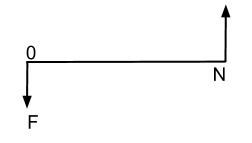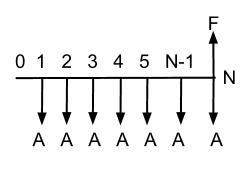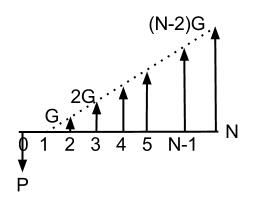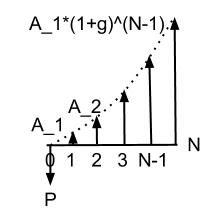Annotated Equations Sheet

# Purpose

The purpose of this page is both to provide the equations provided in the book and on the given equation sheet, (in nice tabular format) AND to mention equations that are not provided on the sheets that can indeed be useful.

# Standard Equation Chart

 Flow Type Factor Notation Formula Excel Command Cash Flow Diagram Common Usages / Examples / Explanation Single Compound Amt (F|P, i, N) (1) \begin{equation} F=P(1+i)^{N} \end{equation} =FV(i, N, P,,0)Single deposit compounded at single rate over time. Single Present Worth (P|F, i, N) (2) \begin{equation} P=F(1+i)^{-N} \end{equation} =PV(i,N,F,,0)Current deposit amount if result is created by periodic compounding at individual rate. Equal Payment Compound Amount (F|A, i, N) (3) \begin{align} F=A\left(\frac{(1+i)^{N}-1}{i}\right) \end{align} =FV(i,N,A,,0)The future value of a series of equal payments, with a specific interest rate Equal Payment Sinking Fund (A|F, i, N) (4) \begin{align} A=F\left(\frac{i}{(1+i)^{N}-1}\right) \end{align} =PMT(i,N,P,F,0)The equal payment amount required to save a given future amount, given rate and future amount Equal Payment Present Worth (P/A, i, N) (5) \begin{align} P=A\left(\frac{(1+i)^{N}-1}{i(1+i)^{N}}\right) \end{align} =PV(i,N,A,,0)The present value of an amount that will be paid off over time using equivalent payments and a specific rate (EX: Calculate mortgage amount via payments) Equal Payment Capital Recovery (A/P, i, N) (6) \begin{align} A=P\left(\frac{i(1+i)^{N}}{(1+i)^{N}-1}\right) \end{align} =PMT(i,N,P)The payment required to pay off a given amount and rate Gradient Series Linear Gradient (P/G, i, N) (7) \begin{align} P=G\left(\frac{(1+i)^{N}-iN-1}{i^{2}(1+i)^{N}}\right) \end{align} UnavailableThe present worth of an amount increasing at a linear rate (every payment = (N-1)*G) Gradient Series Geometric Gradient (P/A_1,g,i,N) (8) \begin{align} P=A_{1}\left(\frac{1-(1+g)^{N}(1+i)^{-N}}{i-g}\right) \end{align} OR (9) \begin{align} P=A_{1}\left(\frac{N}{1+i}\right)\,(if : i = g) \end{align} UnavailableThe present worth of an amount increasing at a geometric rate (every payment = A(1+g)^(N-1))

#### Internal Rate of Return calculations:

1. $A_0$, the present worth of the cash flow in period zero.
2. $A_N\left(1+i\right)^{-N}$, the present worth of the cashflow in period $N$.
(10)
\begin{eqnarray} A_{0}+A_{N}\left(1+i\right)^{-N} & = & 0\\ \left(1+i\right)^{-N} & = & -\frac{A_{0}}{A_{N}}\\ i & = & \left(-\frac{A_{N}}{A_{0}}\right)^{\frac{1}{N}}-1. \end{eqnarray}

#### Tax Calculations:

Actual Rate = Federal Rate + State Rate - (Federal Rate * State Rate)

page revision: 39, last edited: 17 Jun 2016 18:00